# KSEEB Solutions for Class 5 Maths Chapter 10 Data Handling

Students can Download Maths Chapter 10 Data Handling Questions and Answers, Summary, Notes Pdf, KSEEB Solutions for Class 5 Maths helps you to revise the complete Karnataka State Board Syllabus and score more marks in your examinations.

## Karnataka State Syllabus Class 5 Maths Chapter 10 Data Handling

### KSEEB Class 5 Maths Data Handling Ex 10.1

1. Observe the pictograph and answer the questions• Number of Science books read Six
• Number of novels read Eight
• Total number of books read Thirty2. The following pictograph shows the number of cars in 5 villages of a taluk.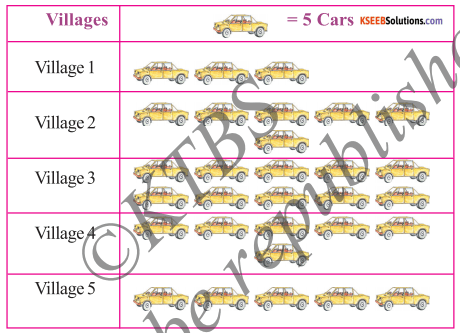Observe the pictograph and answer the following questions:

• Which village has the maximum number of cars? Village 3
• Which village has the minimum number of cars? Village 1
• What is the total number of cars in five villages? 150 cars
• How many more cars are there in village 3 than in village 5? Twenty-five
• Which two villages have the same number of cars? Village 2 & 4

###1. A survey of sale of cars made by an agency for a particular month is an follows: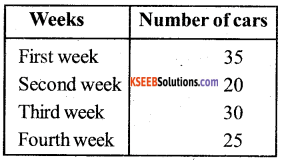Question
Represent this data through a pictograph.
Scale = 5 cars.

Question
In which week were the maximum number of cars sold?
First weekQuestion
In which week were the minimum number of cars sold?
Second week

Question
What is the difference in the number of cars sold in the third and fourth week?
Five years

Question
What is the total number of cars sold in the month?
110 carsQuestion
Arrange the number of cars sold in the descending order along with the week.
Example:
35, 30, 25, 20
First Week

Question 3.
Ramu had 5 pens. 3 erasers, 6 books, 2 pencils and one sharpener in his bag.
Write the data in tubular form and represent the same through a pictograph.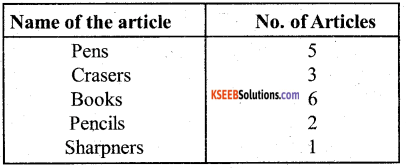4. The number of bulbs manufactured in a week by a factory is given below. Draw a pictograph to represent the data. (Choose a suitable scale)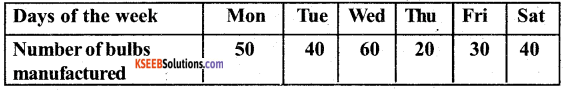Bar GraphsQuestion
What is the information given in the bar graph?
Days against Earnings of flourist in rupeesQuestion
Mention the scale taken.
50 flourist = 1 cm

Question
Mention the earnings on each day.
First day – 300
Second day – 500
Third day – 200
Fourth day – 400
Fifth day – 350

Question
What is the total earnings in five days?
1750 flourist in RupeesQuestion
What is the difference in the amount earned on the second day and the fifth day?
150 flourist in Rupees

Question
Arrange the amount earned on each day in the descending order.
500,400,350,300,200, 2nd day 4th day 5th day ! day 3rd day

2. Study the bar graph and answer the questions given.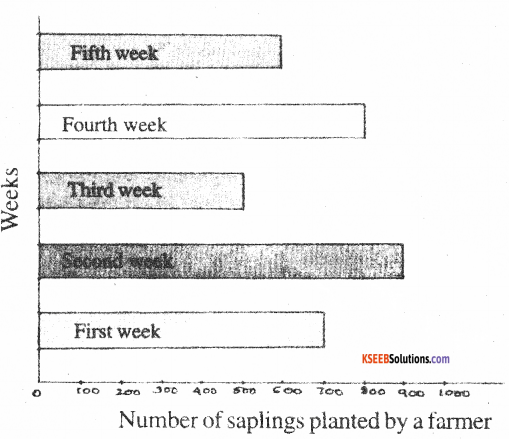Question
What is the information given in the bar graph?
Saplings planted by a farmer against week

Question
Mention the scale taken.
100 Plants = 1 week

Question
Mention the number of saplings planted in each week.
First week – 700
Second week – 900
Third week – 500
Fourth week – 800
Fifth week – 600Question
What is the total number of saplings planted in the five weeks?
3500 saplings

Question
In which week did the farmer plant the maximum number of saplings?
Second week

Question
In which week did he plant the minimum number of saplings?
Third week3. A survey of 120 school students was done to find the activity they prefer to do in their free time.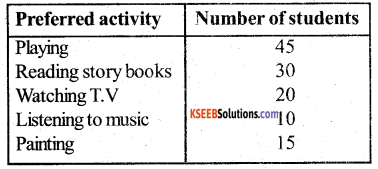Draw a bar graph to illustrate the above data.
Scale: 1 cm= 5 students

Question
Which activity is preferred by most of the students other than playing?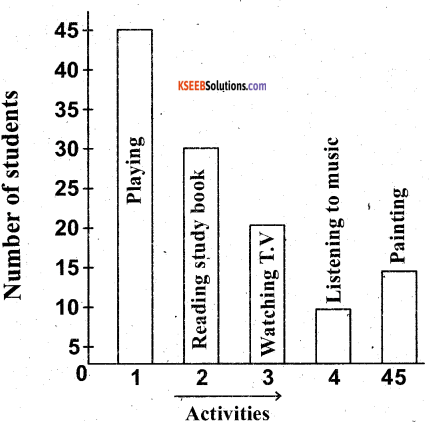4. The number of belts sold by a shopkeeper on six consecutive days of a week is as follows:Draw a bar graph to represent the data.
Scale : 1 cm = 5 belts

Question
What is the total number of belts sold in 6 days?
1755. The number of hours spent by a student on different activities in a day is given below.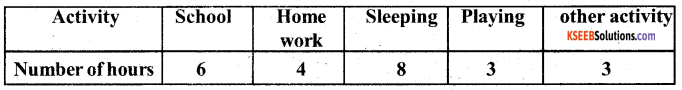Represent the above data by a bar graph.
Scale: 1 cm = 1 hour6. The following table shows the number of bicycles manufactured in a factory during the year 2005 – 2009. Illustrate the data using a bar graph.
(choose a suitable scale)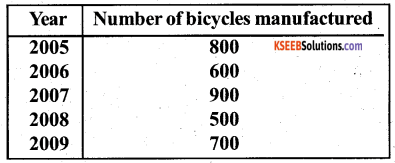Question a.
In which year was the maximum number of bicycles manufactured?